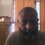# Balancing problem

I tried posting this twice before but could not (sorry for the inconvenience)

This relates to the balancing problem :

5 boxes , 1 box containing coins of wrong weight (correct weight = 10, wrong weight = 11)

Problem : to determine the wrong box in 1 weighing (assuming coins can be removed from boxes and that any weight can be weighed)

Generalized solution :

Let x be the correct weight and y be the wrong weight

We will consider the worst case scenario , ie where x and y are not relatively prime i.e x = 2 and y = 4

1) Choose 1 coin from the first box , 2 from the second box, 4 from the 3rd box and so on (more generally 2^(n-1) from the nth box

2) compute (1+2+4+...2^(N-1))*x , i.e (1+2+....2^(4)) * x This is the correct total weight = T , in our case

31*2 = 62

3) compute actual weight , say W

If box 1 is wrong we get y + 2x + 4x + 8x + 16x = 30x + y 302 + 4 = 64 If box 2 is wrong we get x + 2y + 4x + 8x + 16x = 29x + 2y = 292 + 8 = 66 If box 3 is wrong we get x + 2x + 4y + 8x + 16x = 27x + 4y = 272 + 16 = 70 If box 4 is wrong we get x + 2x + 4x + 8y + 16x = 23x + 8y = 232 + 32 = 78 If box 5 is wrong we get x + 2x + 4x + 8x + 16y = 15x + 16y = 15*2 + 64 = 94

4) compute number of coins from wrong box = |W-T| / |y-x| = 2^(n-1)

If box 1 is wrong we get 2^(n-1) = (64-62) / (4-2) = 2/2 , i.e n=1 If box 2 is wrong we get 2^(n-1) = (66-62) / (4-2) = 2/2 , i.e n=2 If box 3 is wrong we get 2^(n-1) = (70-62) / (4-2) = 2/2 , i.e n= 3 If box 4 is wrong we get 2^(n-1) = (78-62) / (4-2) = 2/2 , i.e n 4 If box 5 is wrong we get 2^(n-1) = (94-62) / (4-2) = 2/2 , i.e n= 5

I suppose this is an abstraction of trying to geometrically determine the center of mass / gravity of a set of points generalizable to least squares etc (if the boxes could be serially placed on a weight, the geometric configuration (sag etc) would determine the wrongly placed weight.

I suppose the above would have applications in spectroscopy / electrophoresis , error correction and other areasNote by Sundar R
7 years, 5 months ago

This discussion board is a place to discuss our Daily Challenges and the math and science related to those challenges. Explanations are more than just a solution — they should explain the steps and thinking strategies that you used to obtain the solution. Comments should further the discussion of math and science.

When posting on Brilliant:

• Use the emojis to react to an explanation, whether you're congratulating a job well done , or just really confused .
• Ask specific questions about the challenge or the steps in somebody's explanation. Well-posed questions can add a lot to the discussion, but posting "I don't understand!" doesn't help anyone.
• Try to contribute something new to the discussion, whether it is an extension, generalization or other idea related to the challenge.

MarkdownAppears as
*italics* or _italics_ italics
**bold** or __bold__ bold
- bulleted- list
• bulleted
• list
1. numbered2. list
1. numbered
2. list
Note: you must add a full line of space before and after lists for them to show up correctly
paragraph 1paragraph 2

paragraph 1

paragraph 2

[example link](https://brilliant.org)example link
> This is a quote
This is a quote
    # I indented these lines
# 4 spaces, and now they show
# up as a code block.

print "hello world"
# I indented these lines
# 4 spaces, and now they show
# up as a code block.

print "hello world"
MathAppears as
Remember to wrap math in $$ ... $$ or $ ... $ to ensure proper formatting.
2 \times 3 $2 \times 3$
2^{34} $2^{34}$
a_{i-1} $a_{i-1}$
\frac{2}{3} $\frac{2}{3}$
\sqrt{2} $\sqrt{2}$
\sum_{i=1}^3 $\sum_{i=1}^3$
\sin \theta $\sin \theta$
\boxed{123} $\boxed{123}$